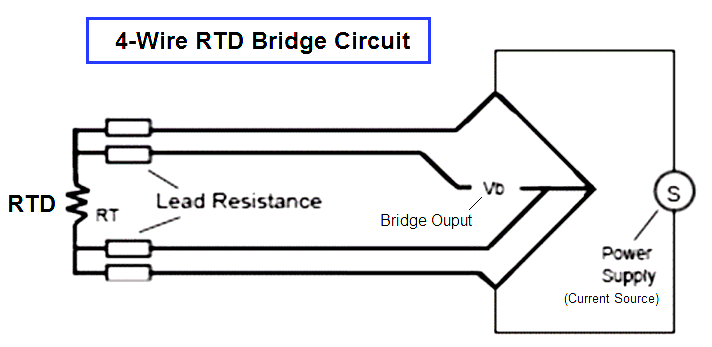# How a 4 Wire RTD Works ?

4 Wire RTD Principle

The most accurate lead wire configuration is the “true” 4-wire configuration. In a true 4- wire configuration, the resistance of the lead wires does not contribute to the resistance of the sensor.

4-wire construction is used primarily where close accuracy is required. In a 4 wire RTD the actual resistance of the lead wires can be determined and removed from the sensor measurement.

The true 4-wire measurement uses the current-potential method. A current of known value (I+) is passed through the sensor along the “current” lead wires. The voltage generated across the sensor is measured using the “potential” lead wires (Vsensor) and the sensor’s resistance is calculated by dividing the measured voltage by the Known current.The 4-wire circuit is a true 4-wire bridge, which works by using wires 1 & 4 to power the circuit and wires 2 & 3 to read. This true bridge method will compensate for any differences in lead wire resistances.

The resistance of the lead wires is not a factor because:

• The value of the current is equal at any point in the circuit. It is independent of the resistance of the lead wire.
• The input impedance of the voltage measurement circuitry is high enough to prevent any significant current flow in the voltage leads. Since no current is flowing, the voltage along the potential leads does not change along their length.

4-wire RTD Bridge CircuitAlso Read: 2 Wire Vs 3 Wire Vs 4 Wire RTD’s